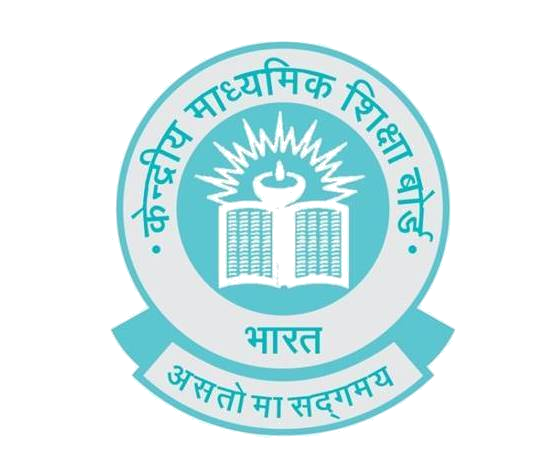# CBSE CLASS 10 MATHS SAMPLE PAPER 2018

## CBSE MATHS SAMPLE PAPER / PRACTICE PAPER 2018Image Credit : www.cbse.nic.in

## Practice Paper – 1

Time Allowed : 3 Hours                                                                                                Maximum Marks : 100
General Instructions :
(i) All questions are compulsory.
(ii) This model test paper contains 30 questions.
(iii) Questions 1 to 6 in section – A are very short answers type questions carrying mark each.
(iv)Questions 7 to 12 in section – B are short answer type questions carrying 2 marks each.
(v) Questions 13 to 22 in section – C are long answer – I type questions carrying 3 marks each.
(vi) Questions 23 to 30 in section – D are long answer – II type questions carrying 4 marks each.
Section A

1. If P( x, y) is equidistant from Q( -2, 5) and R(6, -1) then determine a relationship between x and y.
2. If the nth term of an A.P. is (2n + 1). What is the sum of its first three terms.
3. If P(E) = 0.06, then what is the probability of “not E”.
4. Find the value of x: If cos( 40 + x) = sin 30o.
5. Find the curved surface area of a right circular cone whose radius is 5cm and vertical height is 15cm.
6. What will be the angle of elevation of the sun when the length of a shadow of a vertical Pole is equal to its height.

Section – B

7. Solve  $\frac{4}{x}-3=\frac{5}{2x+3},x\ne 0,x\ne \frac{-3}{2}$
8. Prove $\frac{\mathrm{sin}\theta }{1-\mathrm{cos}\theta }+\frac{\mathrm{tan}\theta }{1+\mathrm{cos}\theta }=\mathrm{sec}\theta \mathrm{cos}ec\theta +\mathrm{cot}\theta$
9. Find the radius of a circle, if the length of tangent from a point at distance of 25cm from the centre of the circle is 24cm.
10. If α and β are the zeros of the polynomial $2{y}^{2}+7y+5$ write the values of α + β + αβ.
11. Construct a line segment AB of length 7cm, Using ruler and compass. Find a point P on AB such that P divides AB in to 3 : 2.
12. Prove that the points (a, b + c), (b, c + a), and (c, a + b) are collinear.

Section – C

13. Solve the quadratic equation $2 x 2 +x−4=0$, by method of completing square.
14. If $tan 2 α=1+2 tan 2 β,$ Prove that $2 sin 2 α=1+ sin 2 β$
15. From a top of a 50m high tower, the angles of depression of the bottom of a pole are observed to be 30o and 45o, respectively. Find the height of the pole. ( √3 =1.73).
16. What least number of the terms of the sequence $17,15 4 5 ,14 3 5 ............$ should be taken, so that the sum is negative.
17. ABC is an isosceles triangle such that AB = AC. D is the mid point of AC, A circle is drawn taking BD as diameter which intersects AB at the point E. Prove that $AE= 1 4 AC$
18. If α and β are the zeroes of the polynomial $2 x 2 −5x+7,$ then find a quadratic polynomial whose zeores are $( 3α+4β ) and ( 4α+3β )$
19. Mallica and Deepica are friends what is the probability that both have
i) different birthdays.
Ii) Same birthday ( ignoring a leap year)
20. Determine the co-ordinates of the centre of a circle passing through the points A(8, 6), B(2, -2) and C(8,-2), Also find the radius of the circle.
21. A survey regarding the heights( in cm) of 50 girls of class X of a school was conducted and the following data was obtained.
Heights(in cm) 120-130 130-140 140-150 150-160 160-170
No. of girls 2 8 12 20 8
Find the mean,meadian and mode of the above data.
22. Solve $ax+by=1, bx+ay= ( a+b ) 2 a 2 + b 2 −1$

Section – D

23. The perimeters of the ends of the frustum of a cone are 207.24cm and 169.56cm. If the height of the frustum be 8cm, find the whole surface of the frustum. [ Use π = 3.14].

24. The sum of five consecutive odd integers is 686. What are the numbers.
25. If $tanA+sinA=m and tanA−sinA=n$ then show that $m 2 − n 2 =4 mn$
26. Solve for x, if possible, $( m 2 + n 2 ) x 2 +( m+n )x+ 1 2 =0$
27. Prove Basic Proportionality Theorem.
28. If P and Q are two points whose, Co-ordinates are $( a t 2 ,2at ) and ( a t 2 , −2a t )$ respectively. S is a point (a, 0) Show that $1 SP + 1 SQ$ is independent of “t”.
29. In given fig. triangle ABC is an obtuse triangle, obtuse angled at B. If AD is perpendicular to CB (produced)Prove that $A C 2 =A B 2 +B C 2 +2BC.BD$
30. It can take 12 hours to fill a swimming pool using two pipes. If the pipe of larger diameter is used for 4 hours and the pipe of smaller diameter for 9 hours, Only half the pool can be filled . How long would it take each pipe to fill the pool separately.

Also Read : CBSE Class10 Sample Papers with Solutions

If You have any Query or suggestion,Please let us know in comment section and you can also contact us via whatsapp on +91 8439619735.

1.Also give their answers along with the,,, solution

1.Contact on Whatsapp number above,we will provide you solution.

2.1.3.4.